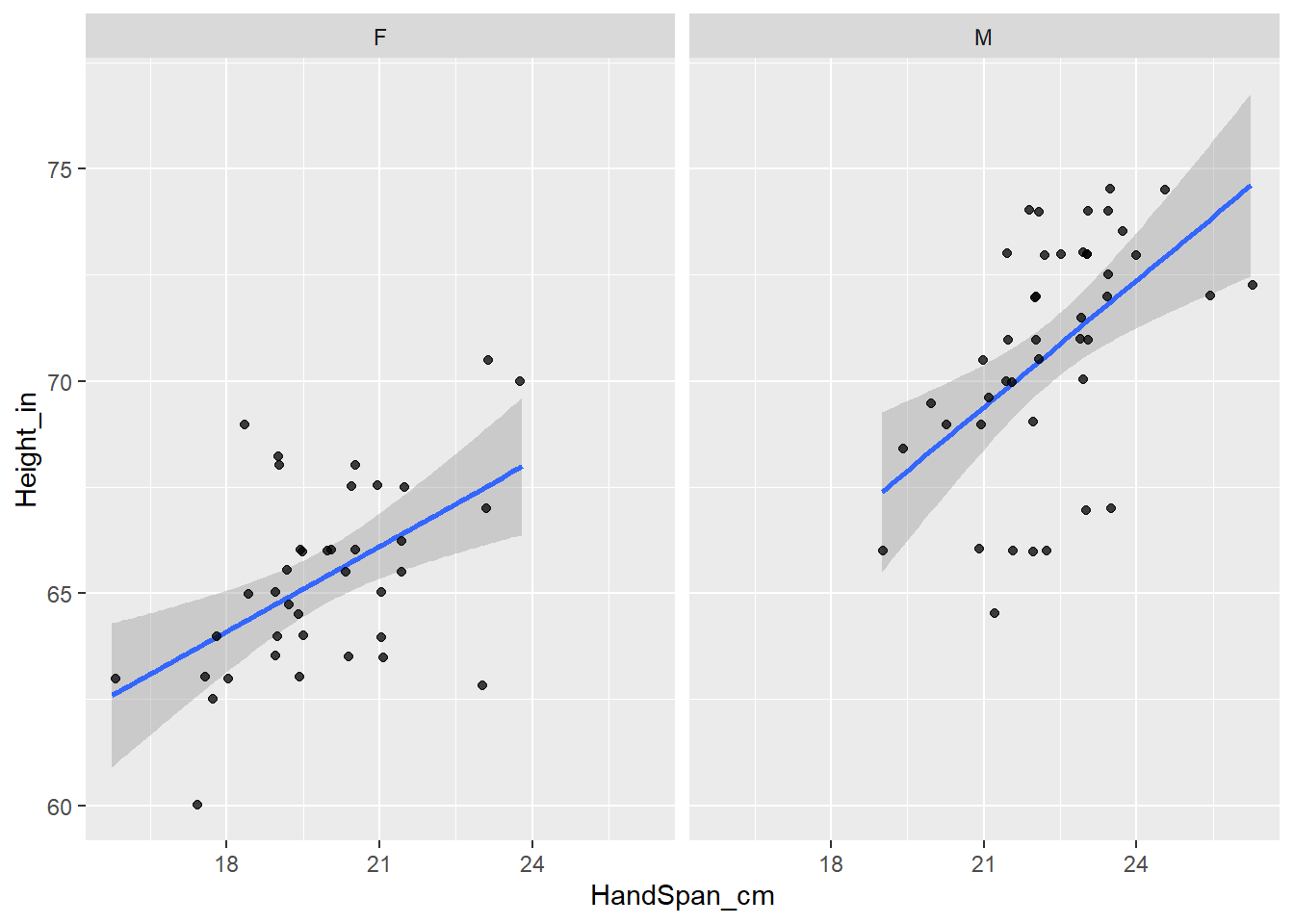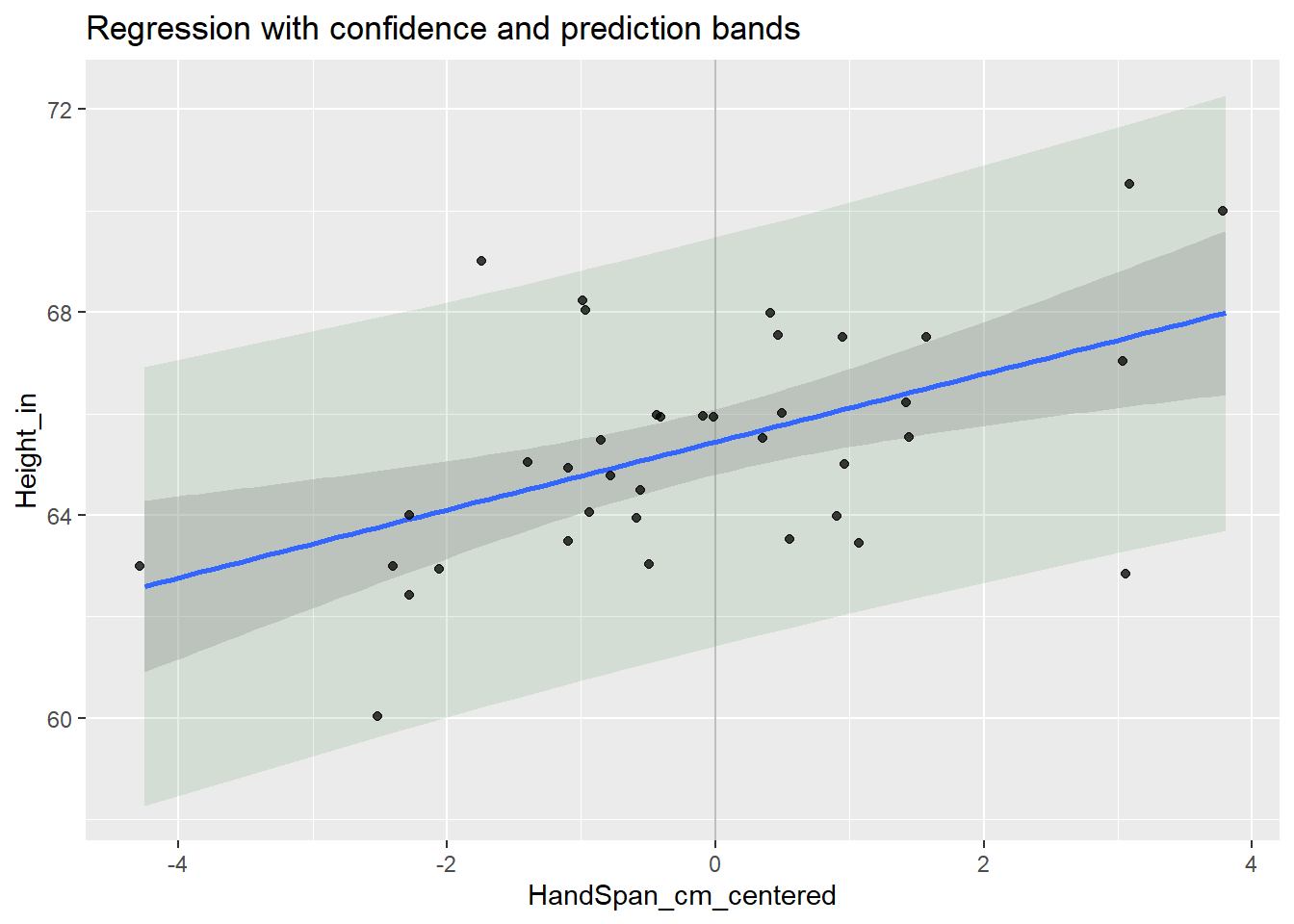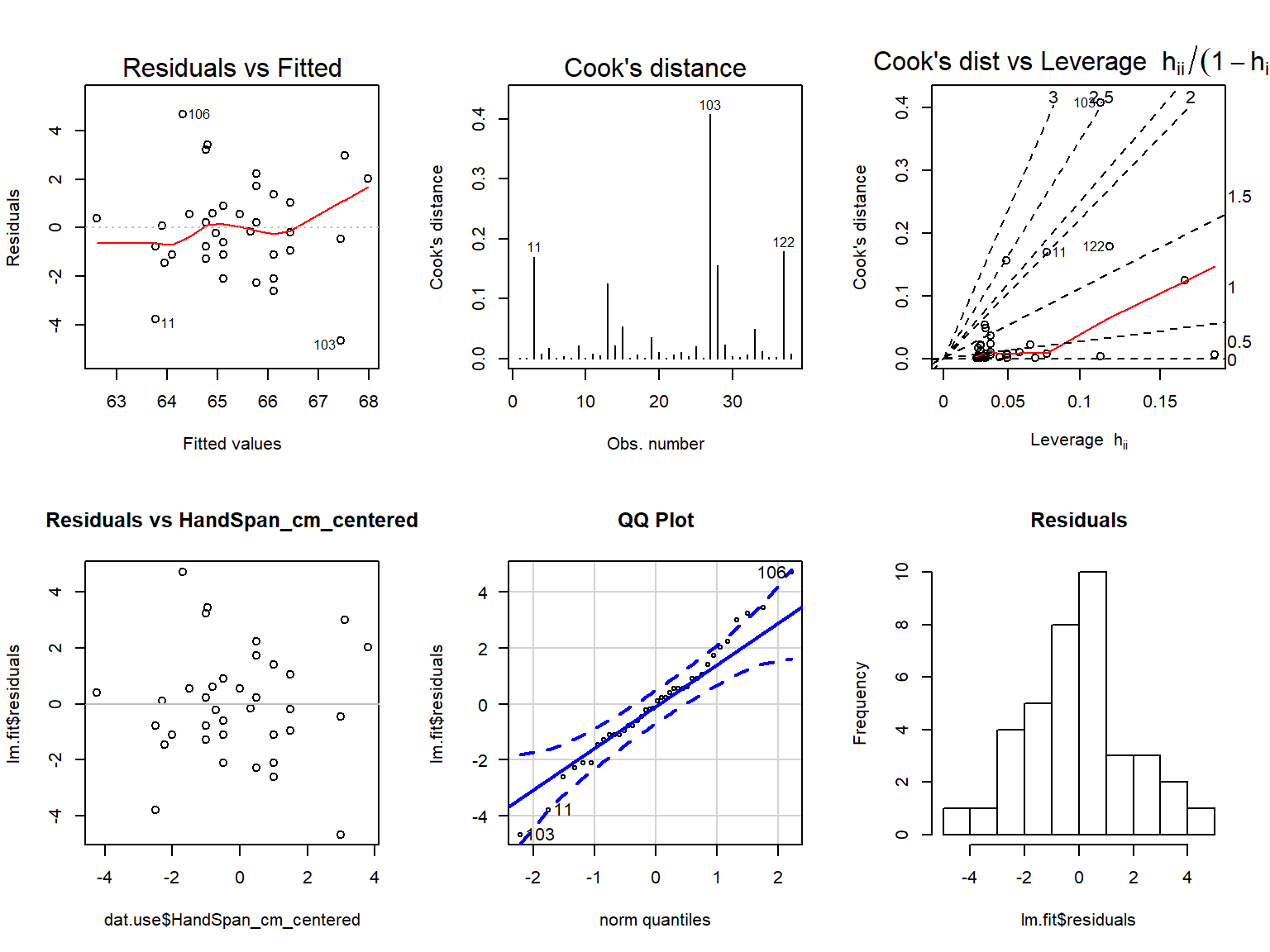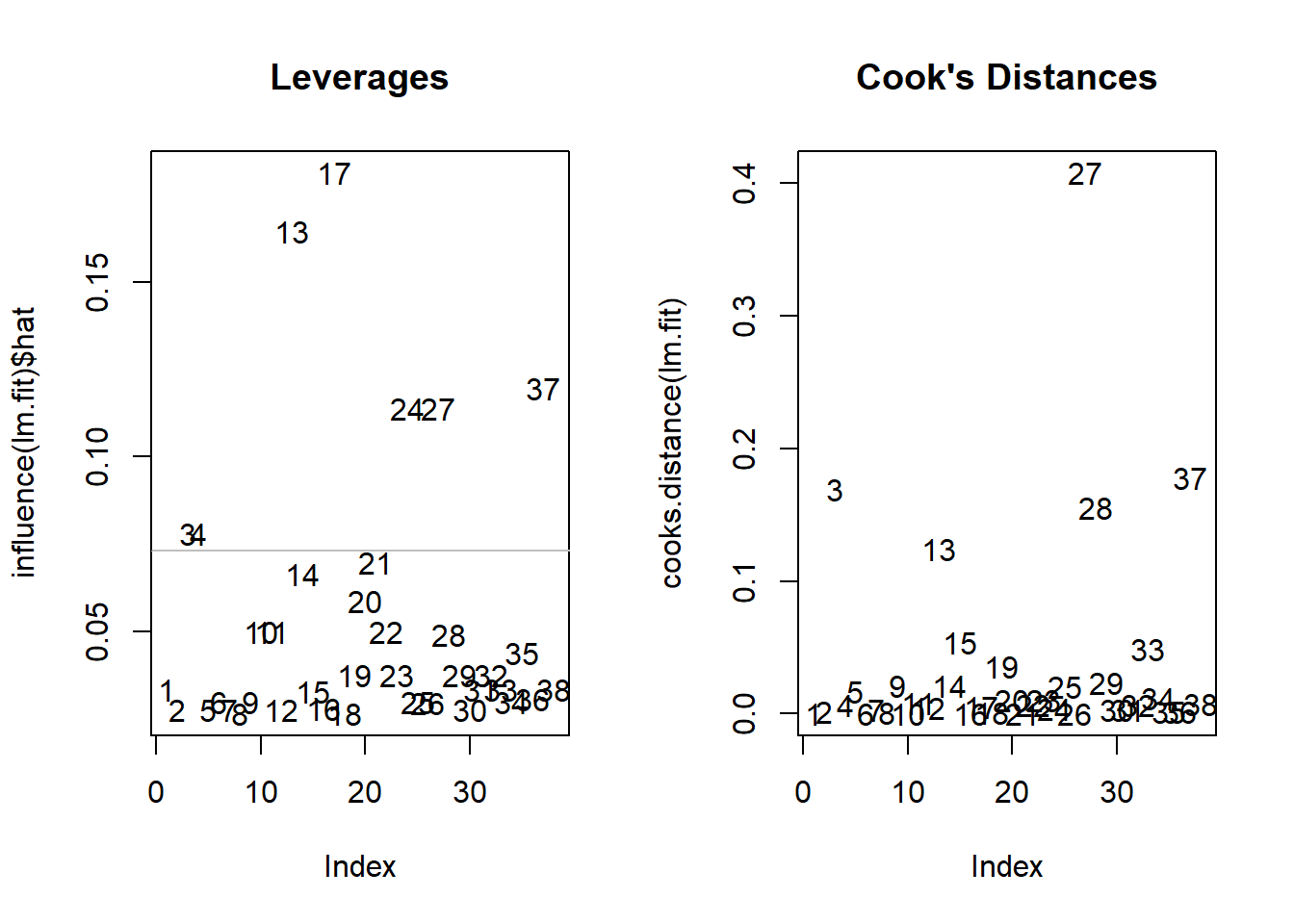Include your answers in this document in the sections below the rubric.

# Rubric

Answer the questions with the data example.

# Height vs Hand Span

We collected this data earlier in the semester.

# install.packages("gsheet")

# Height vs Hand Span
library(gsheet)
dat.hand <- gsheet2tbl(dat.hand.url)
dat.hand <- as.data.frame(dat.hand)
dat.hand <- na.omit(dat.hand)
dat.hand$Gender_M_F <- factor(dat.hand$Gender_M_F, levels = c("F", "M"))

str(dat.hand)
## 'data.frame':    82 obs. of  5 variables:
##  $Table : num 1 1 1 2 2 2 2 2 2 3 ... ##$ Person     : num  1 2 3 1 2 3 4 5 6 1 ...
##  $Gender_M_F : Factor w/ 2 levels "F","M": 2 1 2 1 1 1 2 2 2 1 ... ##$ Height_in  : num  66 65 74.5 64.5 60 63 74 69 67 63 ...
##  $HandSpan_cm: num 21 19 23.5 19.5 17.5 17.5 23.5 21 23 19.5 ... ## - attr(*, "spec")= ## .. cols( ## .. Table = col_double(), ## .. Person = col_double(), ## .. Gender_M_F = col_character(), ## .. Height_in = col_double(), ## .. HandSpan_cm = col_double() ## .. ) ## - attr(*, "na.action")= 'omit' Named int 4 5 6 7 8 9 16 17 18 23 ... ## ..- attr(*, "names")= chr "4" "5" "6" "7" ... Plot data for Height_in vs HandSpan_cm for Females and Males. library(ggplot2) p <- ggplot(dat.hand, aes(x = HandSpan_cm, y = Height_in)) # linear regression fit and confidence bands p <- p + geom_smooth(method = lm, se = TRUE) # jitter a little to uncover duplicate points p <- p + geom_jitter(position = position_jitter(.1), alpha = 0.75) # separate for Females and Males p <- p + facet_wrap(~ Gender_M_F, nrow = 1) print(p)Choose either Females or Males for the remaining analysis. # choose one: dat.use <- subset(dat.hand, (Gender_M_F == "F")) #dat.use <- subset(dat.hand, (Gender_M_F == "M")) Plan: Center the explanatory variable HandSpan_cm, fit a simple linear regression model, check model assumptions, interpret the parameter estimate table, and interpret a confidence and prediction interval. ## Center the explanatory variable HandSpan_cm Recentering the $$x$$-variable doesn’t change the model, but it does provide an interpretation for the intercept of the model. For example, if you interpret the intercept for the regression lines above, it’s the “expected height for a person with a hand span of zero”, but that’s not meaningful. Choose a value to center your data on. A good choice is a nice round number near the mean (or center) of your data. This becomes the value for the interpretation of your intercept. dat.use$HandSpan_cm_centered <- dat.use$HandSpan_cm - 20 ## Fit a simple linear regression model # fit model lm.fit <- lm(Height_in ~ HandSpan_cm_centered, data = dat.use) Here’s the data you’re using for the linear regression, with the regression line and confidence and prediction intervals. library(ggplot2) p <- ggplot(dat.use, aes(x = HandSpan_cm_centered, y = Height_in)) p <- p + geom_vline(xintercept = 0, alpha = 0.25) # prediction bands p <- p + geom_ribbon(aes(ymin = predict(lm.fit, data.frame(HandSpan_cm_centered) , interval = "prediction", level = 0.95)[, 2], ymax = predict(lm.fit, data.frame(HandSpan_cm_centered) , interval = "prediction", level = 0.95)[, 3],) , alpha=0.1, fill="darkgreen") # linear regression fit and confidence bands p <- p + geom_smooth(method = lm, se = TRUE) # jitter a little to uncover duplicate points p <- p + geom_jitter(position = position_jitter(.1), alpha = 0.75) p <- p + labs(title = "Regression with confidence and prediction bands") print(p)## Check model assumptions Present and interpret the residual plots with respect to model assumptions. # plot diagnistics par(mfrow=c(2,3)) plot(lm.fit, which = c(1,4,6)) # residuals vs HandSpan_cm_centered plot(dat.use$HandSpan_cm_centered, lm.fit$residuals, main="Residuals vs HandSpan_cm_centered") # horizontal line at zero abline(h = 0, col = "gray75") # Normality of Residuals library(car) ## Loading required package: carData qqPlot(lm.fit$residuals, las = 1, id = list(n = 3, cex = 1), main="QQ Plot")
## 106 103  11
##  28  27   3
# # residuals vs order of data
# plot(lm.fit$residuals, main="Residuals vs Order of data") # # horizontal line at zero # abline(h = 0, col = "gray75") hist(lm.fit$residuals, breaks=10, main="Residuals")(1 p) If the normality assumption seems to be violated, perform a normality test on the standardized residuals.

(1 p) Do the residuals versus the fitted values and HandSpan_cm_centered values appear random? Or is there a pattern?

## Investigate the relative influence of points

Investigate the leverages and Cook’s Distance. There are recommendations for what’s considered large, for example, a $$3p/n$$ cutoff for large leverages, and a cutoff of 1 for large Cook’s D values. I find it more practical to consider the relative leverage or Cook’s D between all the points and worry when there are only a few that are much more influential than others.

Here’s a plot that duplicates a plot above. Here, the observation number is used as both the plotting point and a label.

# plot diagnistics
par(mfrow=c(1,2))

plot(influence(lm.fit)$hat, main="Leverages", type = "n") text(1:nrow(dat.use), influence(lm.fit)$hat, label=paste(1:nrow(dat.use)))
# horizontal line at zero
abline(h = 3*2/82, col = "gray75")

plot(cooks.distance(lm.fit), main="Cook's Distances", type = "n")
text(1:nrow(dat.use), cooks.distance(lm.fit), label=paste(1:nrow(dat.use)))
# horizontal line at zero
abline(h = 1, col = "gray75")(1 p) Interpret the leverages and Cook’s D values with respect to whether any observations are having undue influence on model fit.

## Interpret the parameter estimate table

Here’s the parameter estimate table.

We’re estimating the $$\beta$$ parameter coefficients in the regression model $$y_i = \beta_0 + \beta_1 x_i + e_i$$.

summary(lm.fit)
##
## Call:
## lm(formula = Height_in ~ HandSpan_cm_centered, data = dat.use)
##
## Residuals:
##     Min      1Q  Median      3Q     Max
## -4.6509 -1.1083 -0.0313  0.8908  4.6937
##
## Coefficients:
##                      Estimate Std. Error t value Pr(>|t|)
## (Intercept)           65.4437     0.3186  205.39  < 2e-16 ***
## HandSpan_cm_centered   0.6691     0.1863    3.59 0.000977 ***
## ---
## Signif. codes:  0 '***' 0.001 '**' 0.01 '*' 0.05 '.' 0.1 ' ' 1
##
## Residual standard error: 1.96 on 36 degrees of freedom
## Multiple R-squared:  0.2637, Adjusted R-squared:  0.2432
## F-statistic: 12.89 on 1 and 36 DF,  p-value: 0.0009768

(1 p) Assuming the model fits well, complete this equation (fill in the $$\beta$$ values with values from the table) with the appropriate numbers from the table above (3 numbers: each beta and the HandSpan centering value).

The regression line is $$\hat{\textrm{Height_in}} = \hat{\beta}_0 + \hat{\beta}_1 \textrm{(HandSpan_cm - 20)}$$.

(2 p) State the hypothesis test related to the slope of the line, indicate the p-value for the test, and state the conclusion.

Words and notation:

• Words:
• Notation: $$H_0:\beta_? = ?$$ vs $$H_A:\beta_? \ne ?$$

(1 p) Interpret the slope coefficient in the context of the model.

(1 p) State and interpret the $$R^2$$ value.

## Interpret a confidence and prediction interval

Below is a 95% confidence interval for the mean (the regression line) and a prediction interval for a new observation at $$\textrm{HandSpan_cm_centered} = -1$$. See how these match up with the plot above.

predict(lm.fit, data.frame(HandSpan_cm_centered = -1)
, interval = "confidence", level = 0.95)
##        fit      lwr      upr
## 1 64.77463 64.04742 65.50184
predict(lm.fit, data.frame(HandSpan_cm_centered = -1)
, interval = "prediction", level = 0.95)
##        fit      lwr      upr
## 1 64.77463 60.73348 68.81578

(1 p) Interpret the CI.

(1 p) Interpret the PI.